# GSEB Solutions Class 12 Physics Chapter 5 Magnetism and Matter

Gujarat Board GSEB Textbook Solutions Class 12 Physics Chapter 5 Magnetism and Matter Textbook Questions and Answers, Additional Important Questions, Notes Pdf.

## Gujarat Board Textbook Solutions Class 12 Physics Chapter 5 Magnetism and Matter

### GSEB Class 12 Physics Magnetism and Matter Text Book Questions and AnswersQuestion 1.
Answer the following questions regarding earth’s magnetism.
(a) A vector needs three quantities for its specification. Name the three independent quantities conventionally used to specify the earth’s magnetic field.

(b) The angle of dip at a location in southern India is about 18°. Would you expect a greater or smaller dip angle in Britain?

(c) If you made a map of magnetic field lines at Melbourne in Australia, would the lines seem to go into the ground or come out of the ground?

(d) In which direction would a compass free to move in the vertical plane point to, if located right on the geomagnetic north or south pole?

(e) The earth’s field, it is claimed, roughly approximates the field due to a dipole of magnetic moment 8 x 10 JT-1 located at its centre. Check the order of magnitude of this number in some way.

(f) Geologists claim that besides the main magnetic N-S poles, there are several local poles on the earth’s surface oriented in different directions. How is such a thing possible at all?

(a) The three quantities are:-

• Magnetic declination
• Angle of dip and
• The horizontal component of the earth’s magnetic field.

(b) Britain is close to magnetic north pole. Therefore, the angle of dip is greater in Britain than in India.  It is about 70°.

(c) The magnetic field lines of the earth could seem to come out of the ground. It is because, Melbourne (Australia) is in Southern hemisphere.

(d) The magnetic compass needle can point in any direction on the geomagnetic north or South pole. It is because the horizontal component of the earth’s field is zero at these points
(∵ The earth’s field is vertical).

(e) Calculate B, from B = $$\frac{\mu_{0} \mathrm{M}}{4 \pi \mathrm{r}^{3}}$$
M = 8 x 1022 JT-1
r = 6.4 x 106 m.
It turns to the value ≈ 0.3 G approximately the horizontal field as the surface.

(f) The earth’s magnetic field is only approximately a dipole field. The local N>S poles may be oriented due to the different deposits of magnetised minerals.Question 2.
(a) The earth’s magnetic field varies from point to point in space. Does it also change with time? If so, on what time scale does it change appreciably?

(b) The earth’s core is known to contain iron. Yet geologists do not regard this as a source of the earth’s magnetism. Why?

(c) The charged currents in the outer conducting regions of the earth’s core are thought to be responsible for earth’s magnetism. What might be the ‘battery’ (i.e., the source of energy) to sustain these currents?

(d) The earth may have even reversed the direction of its field several times during its history of 4 to 5 billion years. How can geologists know about the earth’s field in such distant past?

(e) The earth’s field departs from its dipole shape substantially at large distances (greater than about 30,000 km). What agencies may be responsible for this distortion?

(f) Interstellar space has an extremely weak magnetic field of the order of 10-12 T. Can such a weak field be of any significant consequence? Explain.
[Note: Exercise 2 is meant mainly to arouse your curiosity. Answers to some questions above are tentative or unknown. Brief answers wherever possible are given at the end. For details, you should consult a good text on geomagnetism.]
(a) Yes. Yearly variations or variations in few years. For appreciable change, it may take a few hundred years.

(b) Molten iron is ferromagnetic

(c) Not sharply known, possibly the radioactivity in the interior core.

(d) From the magnetic properties of some rocks.

(e) The motion of ions in ionosphere.

(f) Even if the field is weak, it has cosmological importance. The new particles created in interstellar space will be accelerated by this field.

Question 3.
A short bar magnet placed with its axis at 30° with a uniform external magnetic field of 0. 25 T experiences a torque of magnitude equal to 4.5 x 10-2J. What is the magnitude of the magnetic moment of the magnet?
θ = 30°, B = 0.25 T, τ = 4.5 x 10-2J, m = ?, τ = mBsinθ
∴ m = $$\frac { τ }{ mBsinθ }$$
= $$\frac{4.5 \times 10^{-2}}{0.25 \times \sin 30^{\circ}}$$ = $$\frac{4.5 \times 10^{-2} \times 2}{0.25}$$
= 36.0 x 10-2
= 0.36 Am2 (or) JT-1Question 4.
A short bar magnet of magnetic moment m = 0.32 JT-1 is placed in a uniform magnetic field of 0.15 T. If the bar is free to rotate in the plane of the field, which orientation would correspond to its (a) stable, and (b) unstable equilibrium? What is the potential energy of the magnet in each case?
Solution:
(a) When is parallel to g, the magnet is in stable equilibrium
U = – MB = -0.32 x 0.15 = – 4.8 x 10-2 J

(b) When $$\vec { M }$$ is antiparallel to g, the magnet is in unstable equilibrium.
U = MB = 0.32 x 0.15
= 4.8 x 10-12 J.

Question 5.
A closely wound solenoid of 800 turns and area of cross-section 2.5 x 10-4 m2 carries a current of 3.0 A. Explain the sense in which the solenoid acts like a bar magnet. What is its associated magnetic moment?
Solution:
N = 800, A = 2.5 x 10-4 m2, I = 3.0A, m = ?
m = ANI = 2.5 x 10-4 x 800 x 3.0
= 7.5 x 8 x 102
= 0.600 Am2
The field of solenoid is uniform along the axis, and for short solenoid, it is similar to that of a bar magnet and the polarity depends on the direction of circulation of current.

Question 6.
If the solenoid in Exercise. 5 is free to turn about the vertical direction and a uniform horizontal magnetic field of 0.25 T is applied, what is the magnitude of torque on the solenoid when its axis makes an angle of 30° with the direction of applied field?
Solution:
Here, τ = M B sin θ
M = 0.6 A m2 B = 0.25 T
θ= 30°
∴ τ = 0.6 x 0.25 x sin 30° = 0.075Nm

Question 7.
A bar magnet of magnetic moment 1.5 JT-1 lies aligned with the direction of a uniform magnetic field of 0.22 T.
(a) What is the amount of work required by an external torque to turn the magnet so as to align its magnetic moment:
(i) normal to the field direction
(ii) opposite to the field direction?
(b) What is the torque on the magnet in cases (i) and (ii)?
Solution:
m= 1.5 JT-1 B = 0.22 T
(a) i. mB = 0.330 J
ii. 2mB = 0.66J

(b) i. Maximum = 0.33, tending to turn it towards the direction of the field,
ii. Zero (sin 180° = 0).Question 8.
A closely wound solenoid of 2000 turns and area of cross-section 1.6 x 10-4 m2, carrying a current of 4.0 A, is suspended through its centre allowing it to turn in a horizontal plane.
(a) What is the magnetic moment associated with the solenoid?
(b) What is the force and torque on the solenoid if a uniform horizontal magnetic field of 7.5 x 10-2 T is set up at an angle of 30° with the axis of the solenoid?
Solution:
Number of turns of the solenoid = 2,000
I = 4A
A= 1.6x 10-4m2

(a) The magnetic moment of the solenoid,
M = (IA) x number of turns
= 4 x 1.6 x 10-4 x 2000
= 1.28 Am2

(b) τ-MB sin θ
B = 7.5 x 10-2T
θ = 30°
τ -1.28 x 7.5 x 10-2 x sin30°
= 0.048 Nm
The net force on the solenoid is zero.

Question 9.
A circular coil of 16 turns and radius 10 cm carrying a current of 0.75 A rests with its plane normal to an external field of magnitude 5.0 x 10-2 T. The coil is free to turn about an axis in its plane perpendicular to the field direction. When the coil is turned slightly and released, it oscillates about its stable equilibrium with a frequency of 2.0 s-1. What is the moment of inertia of the coil about its axis of rotation?
Solution:
N= 16, r= 10 cm = 10 x 10-2m, I = 0.75 A
B = 5.0 x 10-2T, υ = 2.0 s-1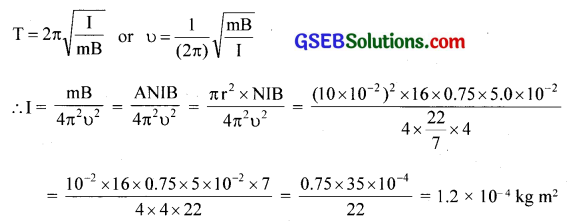Question 10.
A magnetic needle free to rotate in a vertical plane parallel to the magnetic meridian has its north tip pointing down at 22° with the horizontal. The horizontal component of the earth’s magnetic field at the place is known to be 0.35 G. Determine the magnitude of the earth’s magnetic field at the place.
Solution:
θ = 22°, HE = 0.35 x 10-4T, BE = ?
HE = BE cosθ
∴BE = $$\frac{0.35 \times 10^{-4}}{\cos 22^{\circ}}$$
= 0.38 x 10-4 T or 0.38 GQuestion 11.
At a certain location in Africa, a compass points 12° west of the geographic north. The north tip of the magnetic needle of a dip circle placed in the plane of magnetic meridian points 60° above the horizontal. The horizontal component of the earth’s field is measured to be 0.16 G. Specify the direction and magnitude of the earth’s field at the location.
Solution: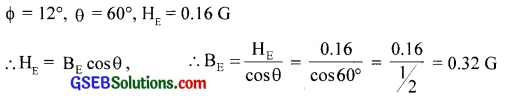The total magnetic field at the place is inclined at 12° towards west from geographic north.

Question 12.
A short bar magnet has a magnetic moment of 0.48 JT-1. Give the direction and magnitude of the magnetic field produced by the magnet at a distance of 10 cm from the centre of the magnet on (a) the axis, (b) the equatorial lines (normal bisector) of the magnet.
Solution:
m = 0.48 JT-1 r = 10 cm
(a) Field on axial line = $$\frac{\mu_{0}}{4 \pi} \frac{2 \mathrm{~m}}{\mathrm{r}^{3}}$$
= $$\frac{10^{-7} \times 2 \times 0.48}{\left(10 \times 10^{-2}\right)^{3}}$$
= $$\frac{10^{-7} \times 2 \times 0.48}{10^{-3}}$$
= 0.96 x 10-4 T, in the direction of in $$\bar { m }$$

(b) Field on equatorial line = $$\frac{\mu_{0}}{4 \pi}$$ x $$\frac{\mathrm{m}}{\mathrm{r}^{3}}$$
= 0.48 x 10-4 T, opposite to $$\bar { m }$$

Question 13.
A short bar magnet placed in a horizontal plane has its axis aligned along the magnetic north-south direction. Null points are found on the axis of the magnet at 14 cm from the center of the magnet. The earth’s magnetic field at the place is 0.36 G and the angle of dip is zero. What is the total magnetic field on the normal bisector of the magnet at the same distance as the null point (i.e., 14 cm) from the centre of the magnet? (At null points, the field due to a magnet is equal and opposite to the horizontal component of the earth’s magnetic field.)
d = 14 cm = 14 x 10-2 m, B = 0.36 G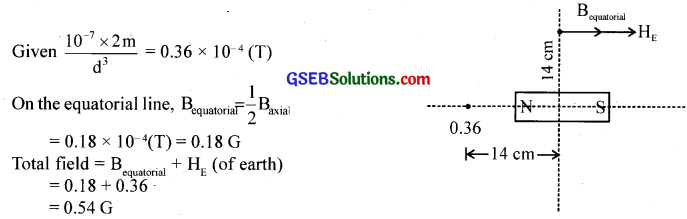Question 14.
If the bar magnet in exercise. 13 is turned around by 180°, where will the new null points be located?
Solution:
On the equatorial line. Let d’ be the corresponding distance. Since the two fields are equal to earth’s field
10-7 x $$\frac{2 \mathrm{~m}}{\mathrm{~d}^{3}}$$
= 10-7 x $$\frac{\mathrm{m}}{\mathrm{d}^{\prime 3}}$$
∴ d‘3 = $$\frac{\mathrm{d}^{3}}{2}$$
d’ = d x 2$$2^{-\frac{1}{3}}$$
= 14 x $$2^{-\frac{1}{3}}$$
= 11.1 cmQuestion 15.
A short bar magnet of magnetic moment 5.25 x 10-2 JT-1 is placed with its axis perpendicular to the earth’s field direction. At what distance from the centre of the magnet, the resultant field is inclined at 45° with earth’s field on (a) its normal bisector and (b) its axis. Magnitude of the earth’s field at the place is given to be 0.42 G. Ignore the length of the magnet in comparison to the distances involved.
Solution:
m = 5.25 x 10-2 JT-1, θ = 90°
BE G = 0.42 x 10-4 T
Since resultant at P makes 45°, tan 45 = $$\frac{\mathrm{B}_{\text {axial }}}{\mathrm{B}_{\mathrm{E}}}$$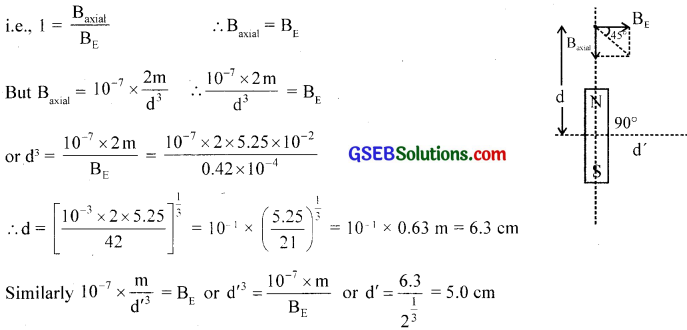Question 16.

1. Why does a paramagnetic sample display greater magnetisation (for the same magnetising field) when cooled?
2. Why is diamagnetism, in contrast, almost independent of temperature?
3. If a toroid uses bismuth for its core, will the field in the core be (slightly) greater or (slightly) less than when the core is empty?
4. Is the permeability of a ferromagnetic material independent of the magnetic field? If not, is it more for lower or higher fields?
5. Magnetic field lines are always nearly normal to the surface of a ferromagnet at every point. (This fact is analogous to the static electric field lines being normal to the surface of a conductor at every point.) Why?
6. Would the maximum possible magnetisation of a paramagnetic sample be of the same order of magnitude as the magnetisation of a ferromagnet?

Solution:

1. The susceptibility X increases.
2. The induced magnetic moment of electrons opposes the external field.
3. Bismuth being diamagnetic, field will be slightly less than the field in its absence.
4. No. it is greater for lower fields.
5. p for ferromagnetic is very large. The lines of force on the surface area and by repulsion take normal shape.
6. Yes. But only under the extremely high magnetising field.

Question 17.
(a) Explain qualitatively on the basis of domain picture the irreversibility in the magnetization curve of a ferromagnet.

(b) The hysteresis loop of a soft iron piece has a much smaller area than that of a carbon steel piece. If the material is to go through repeated cycles of magnetisation, which piece will dissipate greater heat energy?

(c) ‘A system displaying a hysteresis loop such as a ferromagnet, is a device for storing memory?’ Explain the meaning of this statement.

(d) What kind of ferromagnetic material is used for coating magnetic tapes in a cassette player, or for building ‘memory stores’ in a modern computer?

(e) A certain region of space is to be shielded from magnetic fields. Suggest a method.
Solution:
(a) A ferromagnetic material has many small domains of magnetic field. But the magnetic moments of these domains are distributed in random so that there is no magnetism in the unmagnetised state. Under the increasing external magnetic field, the domains merge and magnetism increases until saturation is produced.

On reducing the magnetising field, the merged domains do not split at the rate they merged and hence the retentivity. The domains are not completely randomised even if the external field is brought to zero. This shows the irreversibility in the magnetisation curve of the ferromagnetic material.

(b) Carbon steel piece, because heat lost per cycle is proportional to the area of the hysteresis loop.

(c) Magnetisation of a ferromagnet is not a single-valued function of the magnetising field. Its value for a particular field depends both on the field and also on history of magnetisation (i.e., how many cycles of magnetisation it has gone through, etc.).

In other words, the value of magnetisation is a record or memory of its cycles of magnetisation. If information bits can be made to correspond to these cycles, the system displaying such a hysteresis loop can act as a device for storing information.

(d) Ceramics (specially treated barium iron oxides) also called ferrites.

(e) Surround the region by soft iron rings. Magnetic field lines will be drawn into the rings, and the enclosed space will be free of the magnetic field. But this shielding is only approximate, unlike the perfect electric shielding of a cavity in a conductor placed in an external electric field.Question 18.
A long straight horizontal cable carries a current of 2.5 A in the direction 10° south of west to 10° north of east. The magnetic meridian of the place happens to be 10° west of the geographic meridian. The earth’s magnetic field at the location is 0.33 G, and the angle of dip is zero. Locate the line of neutral points (ignore the thickness of the cable). (At neutral points, magnetic field due to a current-carrying cable is equal and opposite to the horizontal component of earth’s magnetic field.)
Solution:
Since the current is from west to east, magnetic lines of field are in the same direction below but in opposite direction above. At a neutral point, the field of conductor = field of the earth
i.e., $$\frac{\mu_{0} I}{2 \pi a}$$ = B or a = $$\frac{\mu_{0} I}{2 \pi B}$$
I = 2.5 A, B = 0.33 G = 0.33 x 10-4 T
a = $$\frac{4 \pi \times 10^{-7} \times 2.5}{2 \pi \times 0.33 \times 10^{-4}}$$
= $$\frac{5 \times 10^{-3}}{0.33}$$
= $$\frac{5 \times 100 \times 10^{-3}}{33}$$ m
= 1.5 cm.
Parallel to and above the cable at a distance of 1.5 cm.

Question 19.
A telephone cable at a place has four long straight horizontal wires carrying a cur¬rent of 1.0 A in the same direction east to west. The earth’s magnetic field at the place is 0.39 G, and the angle of dip is 35°. The magnetic declination is nearly zero. What are the resultant magnetic fields at points 4.0 cm below the cable?
Solution:
I = 1.0 A, n = 4, BE = 0.39 G
Field at a = 4.0 cm below is B = $$\frac{\mu_{0} I \times n}{2 \pi a}$$
B = BE cos 35
∴ B = $$\frac{4 \pi \times 10^{-7} \times 4 \times 1.0}{2 \pi \times 4 \times 10^{-2}}$$
B = 2 x 10-5 = 0.2 x 10-4 T
This is opposite to BE cos 35° = 0.35 x 10-4 x cos 35° = 0.32 x 10-4
Net field = 0.32 x 10-4 – 0.2 x 10-4 T = 0.12 x 10-4 T = 0.12G
Below the cable
Rh = 0.39 cos 35° – 0.2 = 0.12 G
R = 0.39 sin 35° = 0.22 G
R= $$\sqrt{\mathrm{R}_{\mathrm{h}}^{2}+\mathrm{R}_{\mathrm{v}}^{2}}$$
= 0.22 G
θ = tan-1 $$\frac{R_{v}}{R_{h}}$$ = 62°
Above the cable
Rh = 0.39 cos 35° + 0.2 = 0.52 G
Rv = 0.224 G
R = 0.57 G, θ = 23°Question 20.
A compass needle free to turn in a horizontal plane is placed at the center of a circular coil of 30 turns and a radius of 12 cm. The coil is in a vertical plane making an angle of 45° with the magnetic meridian. When the current in the coil is 0.35 A, the needle points west to east.
(a) Determine the horizontal component of the earth’s magnetic field at the location.
(b) The current in the coil is reversed, and the coil is rotated about its vertical axis by an angle of 90° in the anticlockwise sense looking from above. Predict the declination at the places to be zero.
Solution:
(a) N = 30, r = 12 cm, θ = 45°
I = 0.35A, deflection is along W → E
Field of the coil is directed perpendicular to its plane and is of value
Bcoil = $$\frac{\mu_{0} \mathrm{nI}}{2 \mathrm{r}}$$
The needle stands in the direction of resultant.
Since this is NE,
sin 45° = $$\frac{\mathrm{B}_{\mathrm{E}}}{\mathrm{B}_{\text {coil }}}$$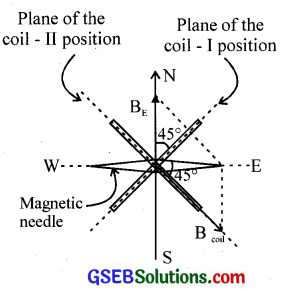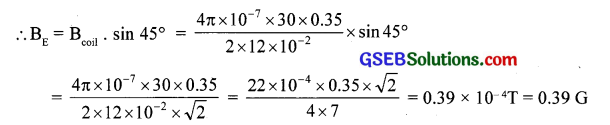(b) As the plane of the coil is rotated through 90° anticlockwise, it takes the 2nd position, on reversing current Bcoil is along with S-W and resultant is along E-W is just opposite to earlier case.

Question 21.
A magnetic dipole is under the influence of two magnetic fields. The angle between the field directions is 60°, and one of the fields has a magnitude of 1.2 x 10-2 T. If the dipole comes to stable equilibrium at an angle of 15° with this field, what is the magnitude of the other field?
Solution: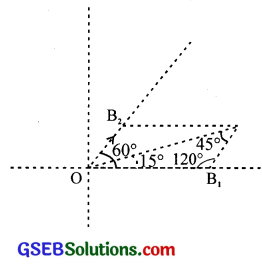Angle between the fields = 60°
Let the fields are B1 and B2, the dipole comes to equilibrium along the direction of the resultant.
Hence from ∆OAC , by sine rule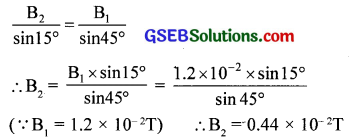Question 22.
A monoenergetic (18 keV) electron beam initially in the horizontal direction is subjected to a horizontal magnetic field of 0.04 G normal to the initial direction. Estimate the up or down deflection of the beam over a distance of 30 cm (me = 9.11 x 10-31 kg). [Note: Data in this exercise are so chosen that the answer will give you an idea of the effect of earth’s magnetic field on the motion of the electron beam from the electron gun to the screen in a TV set.]
Solution: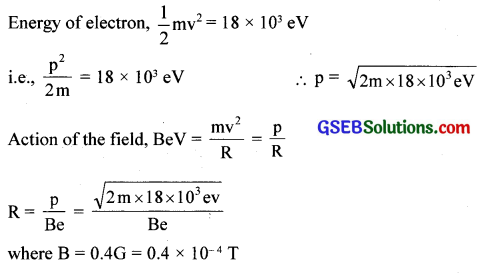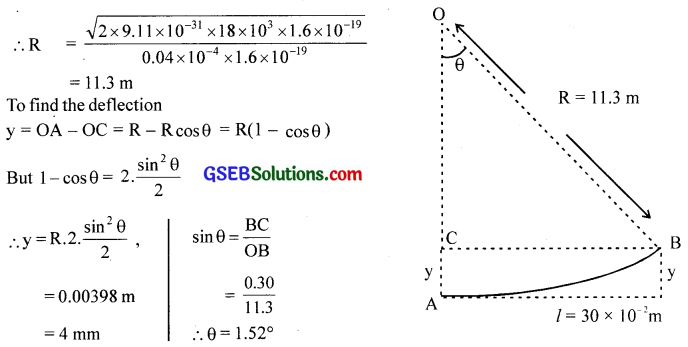Question 23.
A sample of paramagnetic salt contains 2.0 x 1024 atomic dipoles each of dipole moment 1.5 x 1024 JT-1. The sample is placed under a homogeneous magnetic field of 0. 64 T, and cooled to a temperature of 4.2 K. The degree of magnetic saturation achieved is equal to 15%. What is the total dipole moment of the sample for a mag¬netic field of 0.98 T and a temperature of 2.8 K? (Assume Curie’s law)
Solution:
N = 2.0 x 1024
m= 1.5 x 10-23 JT-1
Bex = 0.84 T, T = 4.2 K
% saturation = 15%, ∑Nm = ?
B = 0.98 T,T = 2.8 K
Under the initial conditions, the total magnetic moment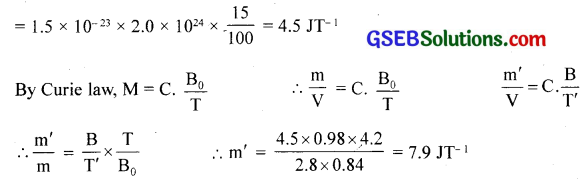Question 24.
A Rowland ring of mean radius 15 cm has 3500 turns of wire wound on a ferromag-netic core of relative permeability 800. What is the magnetic field B in the core for a magnetising current of 1.2 A?
Solution:
R= 15 cm, N = 3500, μr = 800 B = ?, I = 1.2 A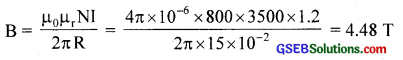Question 25.
The magnetic moment vectors μs and μl associated with the intrinsic spin angular momentum S and orbital angular momentum l, respectively, of an electron, are predicted by quantum theory (and verified experimentally to a high accuracy) to be given by
μs = – $$\frac { e }{ m }$$S
μl = – $$\frac { e }{ 2m }$$l
Which of these relations is in accordance with the result expected classically? Out-line the derivation of the classical result.
Solution:
μ is in accordance with classical theory. It follows easily from the definitions of μl and l:
μl = IA = $$\frac { e }{ T }$$ πr2
l = mvr = m$$\frac{2 \pi r^{2}}{\mathrm{~T}}$$
where r is the radius of the circular orbit which the electron of mass m and charge (- e) completes in time T. Clearly, $$\frac{\mu_{l}}{l}$$
Since charge of the electron is negative (= – e), it is easily seen that 1/4 and 1 are antiparallel, both normal to the plane of the orbit.
Therefore, μl = – $$\frac { e }{ 2m }$$ . 1. $$\frac{\mu_{s}}{S}$$ in contrast to $$\frac{\mu_{l}}{l}$$ is $$\frac { e }{ m }$$, i.e., twice the classically expected value. This latter result (verified experimentally) is an outstanding consequence of modem quantum theory and cannot be obtained classically.

### GSEB Class 12 Physics Magnetism and Matter Additional Important Questions and Answers

Question 1.
Why does the magnet always align in a particular direction?
A freely suspended magnet aligns in a particular direction. This direction is the approximate geographical north-south direction. The magnet is acted by the earth’s natural magnetic field.

Question 2.
What is meant by geographic north pole?
The northernmost part of the earth. (The north endpoint of a diametrical line, perpendicular to equator plane)

Question 3.
What is meant by geographic south pole?
The southernmost part of the earth.Question 4.
The north pole of a freely suspended magnet points towards the geographical north. Why?
The magnetic north is near to the geographic north.

Question 5.
Does the magnetic south pole and geographic north pole coincide?
No.

Question 6.
What is meant by geographic meridian and magnetic meridian?
A vertical plane containing the longitude circle (at the plane) and the axis of rotation of the earth is called the geographic meridian. Similarly, a vertical plane passing through a place and containing the axial line connecting the magnetic north-south poles of earth is called a magnetic meridian.

Question 7.
Do these two meridians coincide anywhere?
Yes. When the two meridians coincide, the declination becomes zero. Places with this property are connected by the line called agonic lines.

Question 8.
When a compass needle is pivoted so that it can rotate in a vertical plane (dip needle), will it align horizontally?
Generally, it will not align horizontally. The dip needle, when properly adjusted, shows an angle with the horizontal called dip.Question 9.
If it is not aligning horizontally, what does it mean?
The net magnetic field is not horizontal.

Question 10.
If the above dip needle is taken to the pole and the magnetic equator, how it will align?
At the pole, the dip is 90° and therefore the dip needle stands vertical. At the equator, the dip is zero. Here the needle stands horizontal.

Question 11.
How can we resolve the earth’s magnetic field at a place into two rectangular components?
If the angle of dip is 0, the horizontal component of total intensity BE is HE = BE cos θ. The other component is Zv = BE sin θ.

Question 12.
What are null points?
The locations where field intensity is zero are called null points.

Question 13.
What are the magnetic field along the axis and equatorial line of a bar magnet?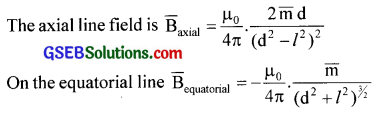Question 14.
Null points are obtained on the axial line when the north pole of the bar magnet is pointing towards the geographical south. Why?
Because earth’s field (magnetic) lines are directed from south to north. The null points cannot be on the equatorial line where the two fields are parallel.Question 15.
Null points are obtained on the equatorial line when the north pole of the bar magnet is pointing towards the geographical north. Why?
The lines of the earth’s magnetic field meet those of the magnet in the opposite direction only on the equatorial line.

Question 16.
A magnetic substance is placed in magnetic fields of different intensities. Does it produce the same effect?
The magnetisation of a material depends on the strength of the external field (or magnetising field). Greater the external field strength, the greater the response.

Question 17.
After magnetizing a substance, by measuring which quantity we can understand the extent to which it is magnetized?
The amount of magnetism attained by a magnetised body is determined by measuring the intensity of magnetization ( $$\bar { M }$$ ). This is defined as a magnetic moment per unit volume.

Question 18.
When we apply an external magnetising field to material, what will be the resultant magnetic field inside it?
Resultant field = external field + field of material.
B = μ0 H ± μ0 M

Question 19.
Is there any relation between B and H?
Yes. The ratio of magnetic induction to magnetising field strength is defined as permeability (μ).
i.e., μ = $$\frac { B }{ H }$$, where μ = μ0 μrQuestion 20.
Is there any relation between M and H?
Yes. The ratio of the intensity of magnetisation to magnetising field strength is defined as the susceptibility (χ).
i.e., $$\frac { M }{ H }$$ = χ

Question 21.
Do all materials have a net magnetic moment in the normal state?
No. For an unmagnetised substance, under a normal state, the net magnetic moment is zero.

Question 22.
What is the reason for the magnetic moment of a material?
The orbital and spin properties of electrons in atoms of the material.

Question 23.
Do all materials behave in the same manner in a magnetic field?
Different magnetic materials like para, Ferro, etc. behave differently to the external fields.Question 24.
Pick the odd one out of the following based on torque.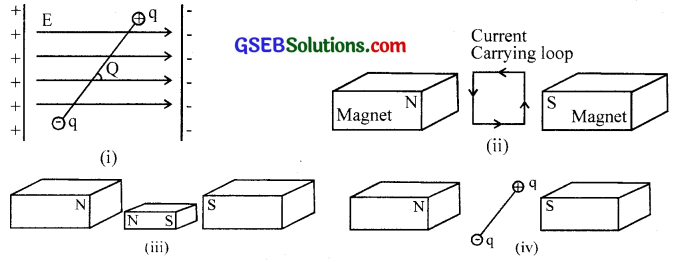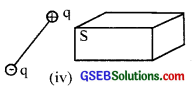Question 25.
The interaction of electric charges q1 and q1, separated by a distance r is F = $$\frac{1}{4 \pi \varepsilon_{0}} \frac{\mathrm{q}_{1} \mathrm{q}_{2}}{\mathrm{r}^{2}}$$. If q1 and q1 are the properties of two magnetic poles, separated by a distance r is F = $$\frac{\mu_{0}}{4 \pi} \frac{\mathrm{p}_{1} \mathrm{p}_{2}}{\mathrm{r}^{2}}$$.
(a) Write two similar properties of these two forces.
(b) Name the quantities p1 p2 and μ0.
(c) Show that ‘p ’ has the unit ampere metre
(a)
i. Both are inverse square law forces
ii. Both are long-range; can be attractive or repulsive.

(b) p1 and p2 are called pole strengths
μ0 – is the property of vacuum (or air) and is called permeability.

(c)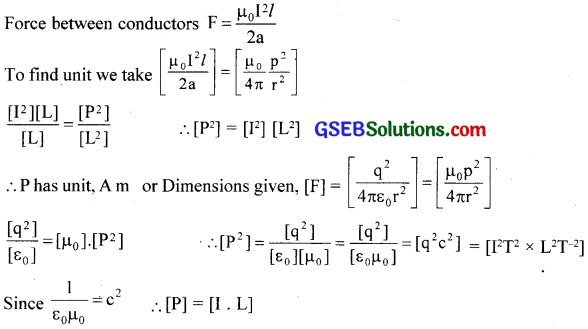Question 26.
A single-pole doesn’t exist. Why?
In a magnet, there is a number of atomic magnets arranged along a line. Each atomic magnet has two poles. So a single-pole can’t exist.Question 27.
Why a freely suspended magnet always points in the north-south direction?
Earth is a huge magnet with its N-pole situated near the geographic south and S-pole near the geographic north. So a freely suspended magnet always points along the north-south direction due to the force of attraction of the opposite poles of the earth’s magnet.

Question 28.
When a bar magnet is dropped from the top of a braiding its magnetism decreases. Why?
The regular arrangement of the molecular magnets in a magnet is disturbed.

Question 29.
Is there any difference between magnetic lines of force and electric lines of force? If your answer is yes, what is that difference?
Yes. Even in constant field conditions, magnetic lines of force are closed.

Question 30.
Magnetic lines of force never intersect each other. What is the reason?
The direction of the magnetic field is given by the direction of the tangent drawn to the line of force. If two lines of force intersect, at the intersecting point there will be two directions for the field. This is not possible since the field is unidirectional.Question 31.
A bar magnet of length 21 and pole strength m is placed in a uniform magnetic field B, inclined at angle ‘0’ with the field.
(a) What is the force acting on each pole?
(b) Why the magnet gets a rotating effect?
(a) Force, F = mB where ‘m’ is the pole strength and B is the magnetic induction.
(b) Because it is acted by a torque.

Question 32.
Show how a current loop acts as a magnetic dipole. Arrive at an expression for the magnetic dipole moment.
Consider a plane loop of wire carrying a current ‘I’ as shown in the figure. Looking at the lower face, the current is clockwise and so it has a south polarity. Thus the current loop behaves as a magnetic dipole. If A is the area of the plane of the loop, then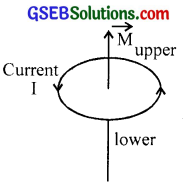dipole moment M = IA
For n such turns, M = nIA
S.I unit of M is A.m2

Question 33.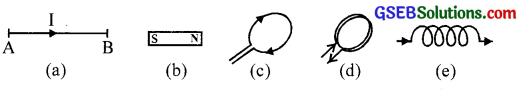From the above collection, two bodies show almost identical field patterns.
(a) Which are they?
(b) Draw the lines of force produced by them.
(c) Identify the cause producing the field in each.
(a) The bar magnet and solenoid ((b) and (e))
(b)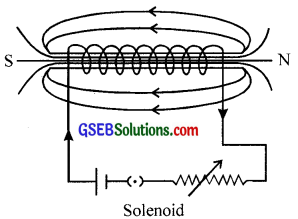(c) Molecular magnets in bar magnet and current loops (the individual turns)

Question 34.
In what way Gauss’s theorem in magnetostatics differs from the one in electrostatics? What conclusion do you draw from this?
The total flux passing through a closed surface is zero in the magnetic field unlike that of electric flux in an electrostatic field. No, monopole exists in magnetism

Question 35.
What do you know about the following terms?
(a) Isogonic line and Agonic line
(b) Isoclinic line and Aclinic line
(c) Isodynamic line
(a) Isogonic lines – the lines joining the place of the same magnetic declination.
Agonic lines – the lines passing through places of zero declination

(b) Isoclinic lines – the lines joining the places of the same dip.
Aclinic lines – the lines passing through places of zero dip

(c) Isodynamic – the lines of the same horizontal intensity.Question 36.
Show that the magnetic moment of an atom is M = $$\frac { 1 }{ 2 }$$eωr2, where e the charge of an electron, ω – the angular speed of electron and r – the radius of electron orbit.
The electrons are revolving around the nucleus of an atom. If ‘e’ is the charge of an electron and T its period of revolution, then
Current I = $$\frac { e }{ T }$$ = $$\frac { eω }{ 2π }$$.
Since T = $$\frac { 2π }{ ω }$$, ω is the angular velocity.
If A = πr2, is the area of the orbit of radius ‘r’, then a magnetic moment of the atom,
M = IA = $$\frac { eω }{ 2π }$$.πr2 = $$\frac { 1 }{ 2 }$$eωr2.

Question 37.
According to Bohr’s postulate, angular momentum of an electron is mvr = $$\frac { nh }{ 2π }$$. Using this formula shows that the magnetic moment of the atom is M = nμB. Here what is pB and what is its value?
According to Bohr’s postulate, the angular momentum of an electron, mvr = $$\frac { nh }{ 2π }$$
where m – mass of the electron, v – velocity of the electron, h – Planck’s constant and n -1, 2, etc, the no. of orbits.
Since v = rω, we have mωr2 = $$\frac { nh }{ 2π }$$ ∴ M = $$\frac { 1 }{ 2 }$$eωr2 = $$\frac { 1 }{ 2 }$$ e.$$\frac { nh }{ 2πm }$$ = n.$$\frac { eh }{ 4πm }$$
ie., M= nμB where $$\frac { eh }{ 4πm }$$ = μB, called Bohr Magneton. Bohr magneton is the unit of atomic magnetic dipole moment.Question 38.
A rod of a magnetic material moves with very small velocity v as shown in Figure below, through a uniform magnetic field. Draw how the magnetic lines of force take shape if the magnetic material is (i) Ferromagnetic (ii) Paramagnetic and (iii) Diamagnetic.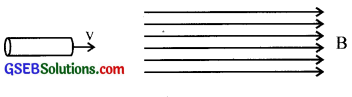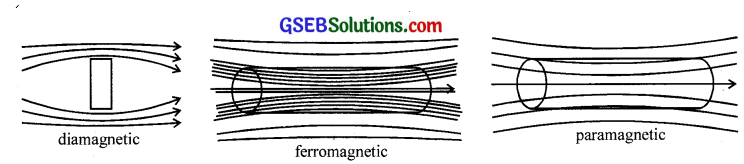Question 39.
The following terms find importance in magnetism. Explain them
(a) Magnetic permeability
(b) Magnetic intensity (H)
(c) Intensity of magnetisation (I)
(d) Magnetic susceptibility (χm)
(a) Magnetic permeability is the ratio of magnetic induction to magnetising field strength.
permeability, μ = $$\frac { B }{ H }$$

(b) It is the field produced by the Amperian current. If n is the number of turns of a solenoid and I – the current through it, then H = nI. It is the magnetic field that magnetises the medium and is called magnetic intensity.

(c) Intensity of magnetisation is defined as the magnetic moment per unit volume.
I = $$\frac { M }{ V }$$, M – magnetic moment, V – volume

(d) The ratio of intensity of magnetisation to the magnetising field strength is defined as magnetic susceptibility( χ )
χ = $$\frac { I }{ H }$$

Question 40.
The figure shows a solenoid carrying a current of I – ampere. Its area is ‘A ’ and the number of turns n.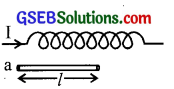(a) What is the flux density of the solenoidal field inside?
(b) If magnetic material in the form of a rod of area ‘a’
(a << A) is inserted into the solenoid; what is the magnetising field strength?
(c) Find the total flux density inside the specimen
(d) Derive expressions for susceptibility and relative permeability.
(e) Classify magnetic substances based on permeability, susceptibility, and total permeability. Give atleast two examples for each type.
(a) B0 = μ0 nI (taking n number of tums/unit length)

(b) H = nI

(c) B = B0 + Bm.
Total flux density B. Total flux density free space = B0
B0 – induction due to magnetisation of the material.

(d) Susceptibility, χm = 7.
μH = μ0 H + μ0 I ∴ μ = μ0(I + $$\frac { I }{ H }$$) = μ0(I + χ)
Let μR be the relative permeability, then μRμ0 = μ0(I+χ)
∴ μr = (I+χ)

(e) Diamagnetic -1 ≤ χ < 0;0 < μr < 1, μ< μ0
Paramagnetic 0 < χ < 1; μr > 1, μ > μ0
Ferromagnetic χ >> 1; μr >> 1, μ >> μ0
Examples : diamagnetic – Bismuth, lead, gold, mercury, silver etc.
Paramagnetic – Aluminium, chromium, oxygen, etc.
Ferromagnetic – Iron, cobalt, nickel, etc.Question 41.
The relation between μr and is χm is μr = I + χm. How will you arrive at this relation? Explain.
We have total magnetic induction B = B0 + Bm
where B0 – magnetic induction in free space.
Bm – magnetic induction due to magnetisation of the material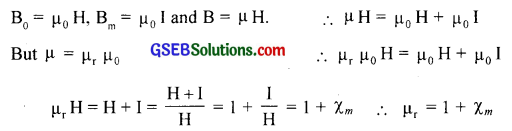Question 42.
On the basis of magnetic properties, the substances are classified into three.
(a) What are these three classifications?
(b) Explain each of them.
(c) Give three examples for each.
(a) On the basis of magnetic properties, different materials are classified into

• Diamagnetic substances
• Paramagnetic substances
• Ferromagnetic substances

(b) On the basis of magnetic properties, different materials are classified into

• Dia magnetic substances
• Paramagnetic substances
• Ferromagnetic substances

Diamagnetic substances are those substances in which the individual atom or molecule or ion does not possess any net magnetic moment of their own. These substances experience a very weak repulsive force in a magnetic field.
Example: Antimony, Bismuth, Copper, Gold, Quartz, Mercury, Lead, Water, Alcohol, Air, Hydrogen etc.

Paramagnetic substances are those substances in which the individual atom or molecule or ion possesses a net non-zero magnetic moment of their own. These substances experience a weak attractive force in a magnetic field.
Example: Aluminium, Platinum, Chromium, Manganese, Sodium, Copper chloride, Crown glass, Liquid oxygen, Salt solutions of iron and nickel.

Ferromagnetic substances are those substances in which each individual atom or molecule or ion possesses a non-zero magnetic moment. These substances experience a very strong force of attraction in a magnetic field.
Example: Iron, Nickel, Cobalt, etc.

(c) Based on the values and signs of susceptibility and permeability, we classify materials as diamagnetic, paramagnetic, and (or) ferromagnetic. It is now better to have a glance at the table.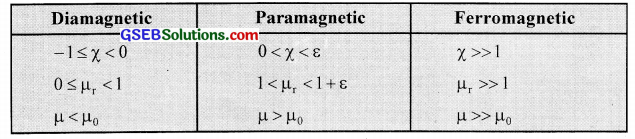Question 43.
Is there any importance for the Curie point? Explain.
When the temperature rises, the susceptibility of ferromagnetic substances decreases. At a certain temperature, its ferromagnetic property completely loses and becomes paramagnetic in nature. The temperature at which the transition from Ferro to para takes place is called Curie point or Curie temperature, e.g. For iron, Curie temp is about 1000 K, for Cobalt – about 1400 K and for Nickel about 631 K.Question 44.
A (hypothetical) bar magnet (AB) is cut into two equal parts. One part is now kept over the other, so that pole C2 is above C1 If M is the magnetic moment of the original magnet, what would be the magnetic moment of the combination so formed?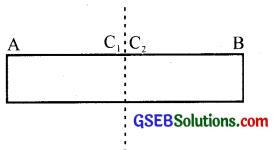Zero

Question 45.
Define the S.I unit of the magnetic field. “A charge moving at right angles to a uniform magnetic, the field does not undergo a change in kinetic energy.” Why?
Tesla is the SI unit of the magnetic field. The magnetic field at a point is one Tesla if a charge of one coulomb while moving perpendicular to the magnetic field with a velocity of 1 ms-1 experiences a force of 1 newton at that point.

The force on a moving charged particle in a magnetic field is perpendicular to its direction of motion. So work done on the charged particle by the magnetic force is zero. Hence the kinetic energy of a charged particle in a magnetic field remains unchanged.

Question 46.
How does the (i) pole strength and (ii) magnetic moment of each part of a bar magnet change if it is cut into two equal pieces along its length?
Each piece is a magnet with reduced pole strength and reduced magnetic moment.Question 47.
(a) Where on the earth’s surface is the value of the vertical component of the earth’s magnetic field zero?
(b) The horizontal component of the earth’s magnetic field at a given place is 0.4 x 10-4 Wb/m2 and the angle of dip is 30°. Calculate the value of (i) vertical component (ii) the total intensity of the earth s magnetic field.
(a) At the equator, the value of the vertical component of the earth’s magnetic field is zero.

(b) i. ZE = B sin I = 0.4 x 10-4 x tan 30° = 0.23 x 10-4 Wbm-2
ii. Total intensity of earth’s magnetic field, BE
BE = $$\frac{\mathrm{H}_{\mathrm{E}}}{\cos \mathrm{I}}$$
= $$\frac{0.4 \times 10^{-4}}{\cos 30^{\circ}}$$
= 0.46 x 10-4Wbm-2

Question 48.
A uniform magnetic field gets modified as shown when two specimens X and Y are placed in it.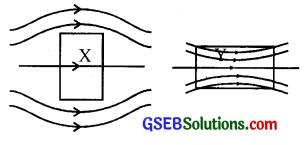i. Identify the two specimens X and Y
ii. State the reason for the behaviour of the field lines in X and Y.
i. X is a diamagnetic substance.
Y is a paramagnetic substance.

ii. When a diamagnetic bar is placed in an external field, the field lines are repelled or expelled and the field inside the material is reduced. When a paramagnetic bar is placed in an external field, the field lines get concentrated inside the material and the field is increased.

Question 49.
How will a dia, para, and ferromagnetic material behave when kept in a nonuniform external magnetic field? Give one example of each of these materials.